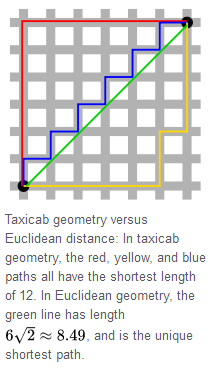# Deforming a Circle

Geometry Level 2In taxicab geometry, Euclidean distance for a point $(x_1,y_1)$ and a point $(x_2,y_2)$ $d=\sqrt {(x_1-x_2)^2+(y_1-y_2)^2}$ is replaced by Manhattan distance $d=|x_1-x_2|+|y_1-y_2|$ and the shape of circles may change as well.

What is the shape of taxicab circles?

×